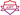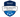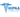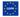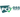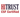# PHP Type Juggling

by Anthony Ralston | August 12, 2020 | Blog

Type juggling is an expected functionality of PHP when leveraging loose comparisons. However, it can be used to subvert intended operations. In this blog, we will discuss why type juggling occurs, what are the potential impacts, and why we should use strict comparisons when developing applications in PHP.

## Equal vs Identical

In PHP, there is a big difference between “==” (Equal or called “Loose Comparison”) and “===” (Identical or called “Strict Comparisons”). Equal will check to see if the variables are equal to each other after type juggling occurs. Identical checks to see if the variables are equal and are the same type.

#### Example of Loose Comparison

php > var_dump(‘0’ == 0);
bool(true)
Notes: This is true because type juggling changed the string 0 to the integer of zero and they are equal.#### Example of Strict Comparison

php > var_dump(‘0’ === 0);
bool(false)
Notes: This is false because they have two different types. One is a string and one being an integer. That means it will not be identical or it is false.## Types

#### String

Any string of characters, specified by single quotes, double quotes, heredoc syntax, or nowdoc syntax.

• single quote:
\$string = ‘This is the string’
• double quote:
\$string = “This is the string”
• heredoc syntax:
\$string = <<<EOT
This is the string
EOT;
• nowdoc syntax:
\$string = <<<‘EOT’
This is the string
EOT;

#### Integer

An integer is a number on the number line (-2, -1, 0, 1, 2,). They can be decimal, octal, hexadecimal, binary, or _ decimal (as of PHP 7.4.0) numbers.

##### Float

Floats or “real numbers” are numbers with the below syn taxes
\$a = 1.234;
\$b = 1.2e3;
\$c = 7E-10;
\$d = 1_234.567; // as of PHP 7.4.0

## PHP Type Juggling

PHP does not support explicit type definition in variable declarations. This means that type is decided based on the context in which the variable is used.

`\$foo = "1"; // \$foo is string (ASCII 49)`

`\$foo *= 2; // \$foo is now an integer (2)`

`\$foo = \$foo * 1.3; // \$foo is now a float (2.6)`

`\$foo = 5 * "10 Little Piggies"; // \$foo is integer (50)`

`\$foo = 5 * "10 Small Pigs"; // \$foo is integer (50)`

## Magic Hash

“e” is a numerical constant and when you have a hash like 1e1 this would equal 10 or 1 and 1 zero. Likewise, if you had a 5e10, it would be a 5 and 10 zeros.

php> var_dump(5e10 == 50000000000);
bool(true)
Notes: with type juggling we are assuming that the hash or string is represented as a string, however in PHP the type is determined by the context in which the variable is used.

php> var_dump(‘5e10qwe’ == 50000000000);
bool(true)
php > var_dump(‘5e10qwe’ == ‘50000000000’);
bool(false)

As we can see when the string is compared with an integer the string is treated as an integer. This means that if a hash starts with 0e123 it will = 0.

Note: This will more likely happen in MD5 and SHA1 hash values.

References

https://www.php.net/manual/en/language.types.type-juggling.php

https://www.php.net/manual/en/language.types.string.php

https://www.php.net/manual/en/language.types.integer.php

https://www.php.net/manual/en/language.types.float.php

https://owasp.org/www-pdf-archive/PHPMagicTricks-TypeJuggling.pdf